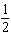4.OA.2.C1 Multiply or divide to solve word problems
 Name:    4.OA.2.C1 Multiply or divide to solve word problems

Multiple Choice
Identify the choice that best completes the statement or answers the question.

1.

Jenna ran ½ mile at field day.  Katie ran 4 times farther than Jenna.  How far did Katie run?
 a. 5 miles c. 3 miles b. 4 miles d. 2 miles

2.

860 = 430 X  n   Solve for n.
 a. n = 2 c. n = 5 b. n = 3 d. n = 4

3.

Which equation represents the comparison:  seven times more students attend Roosevelt School as attend Edison School.  Eighty students attend Edison School.  How many students attend Roosevelt School?
 a. 7 + 80 = 87 c. 80 - 7 = 73 b. 5 X 80 = 400 d. 7 X 80 = 560

4.

The 4th grade class gathered data to find out how many students owned cats and dogs.  The class discovered that 8 times more students owned cats as owned dogs.  Four hundred sixteen students owned cats.  How many students own dogs?
 a. 400 c. 52 b. 408 d. 204

5.

 a. 5 c. 2 b. 3 d.6.

Jose joined the swim team.  He swims 15 laps at every practice.  Jose practices 5 times per week.  How many laps does Jose swim per week?
 a. 20 laps c. 45 laps b. 75 laps d. 30 laps

7.

Greg went to the grocery store to buy bread and chocolate chips.  He noticed the chocolate chips cost five times more than the bread.  If the chocolate chips cost \$5.55, how much did the bread cost?
 a. \$2.50 c. \$5.60 b. \$10.10 d. \$1.11

8.

The fourth grade class is earning money for a field trip.  They earned \$250 selling greeting cards.  They earned three times as much money selling popsicles after lunch.  How much did they earn selling popsicles?
 a. \$500 c. \$253 b. \$750 d. \$300

9.

David has earned 1/3 of the money he needs to buy a new skateboard.  If the skateboard costs \$96, how much money has David earned?
 a. \$35 c. \$45 b. \$48 d. \$32

10.

1.200 = 4 X n  Solve for n.
 a. n = 300 c. n = 1,204 b. n = 200 d. n = 400